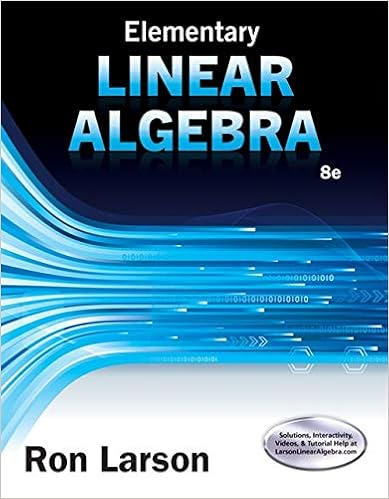# vectors - EXPERIMENT 2 Addition of Vectors OBJECTIVES When...

• Lab Report
• coursefinder123
• 6
• 25% (4) 1 out of 4 people found this document helpful

This preview shows page 1 - 2 out of 6 pages.

##### We have textbook solutions for you!
The document you are viewing contains questions related to this textbook.The document you are viewing contains questions related to this textbook.
Chapter 4 / Exercise 60
Elementary Linear Algebra
LarsonExpert Verified
EXPERIMENT 2 - Addition of Vectors OBJECTIVESWhen a number of vectors passing through the same point act on an object, it may be replaced with a single vector which is called the resultant or the sum. The resultant therefore is a single vector which is equal to the effect produced by the several forces acting on the body.The objectives of this lab are:1. To obtain the resultant of a number of vectors acting on a body. This will be accomplished by finding the magnitude and direction of a single vector necessary to make a ring centered around a pin located in the center of a turntable when placed on a force table along with the vectors it is to replace.A trial and error method will be used in the laboratory experiment. 2. To obtain the resultant of the same forces by drawing a vector diagram to scale to find its magnitude and direction.3. Determination of the resultant using the analytical method, including the law of cosines for the magnitude and the law of sines to find its direction in one instance. The components method will also be used.MATERIALS1. Force table5. Strings for suspending the masses2. Four weight holders6. A ring3. Four pulleys7. A metal pin 4. Slotted weights8. A protractor9. Sheets of plain paper or graph paper.
##### We have textbook solutions for you!
The document you are viewing contains questions related to this textbook.The document you are viewing contains questions related to this textbook.
Chapter 4 / Exercise 60
Elementary Linear Algebra
LarsonExpert Verified
•••